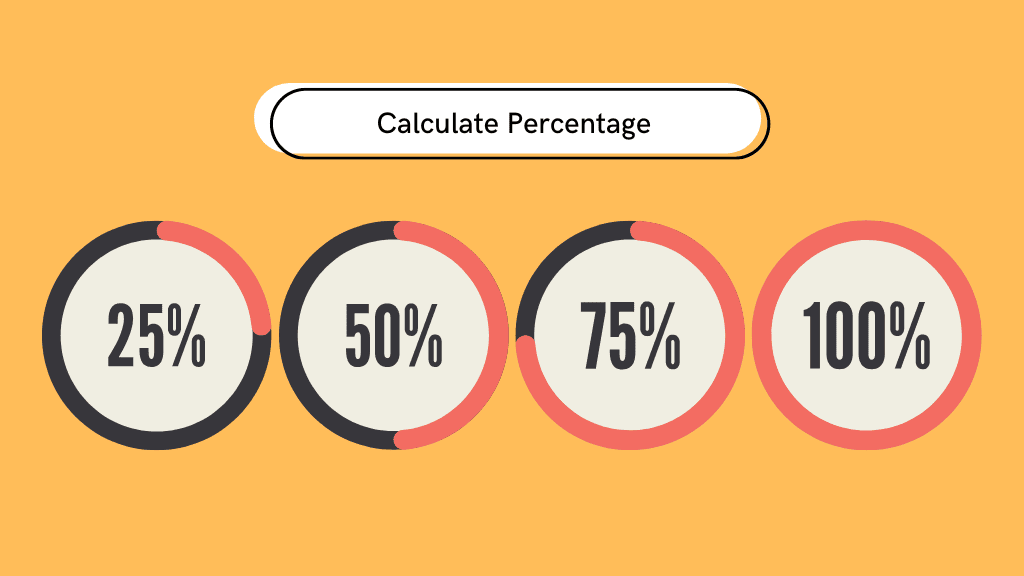# Calculation of percentage (%) online with formula## How to calculate a percentage?

What is
of the total

The number

increased by

The number

increased by

The number

decreased by

The number

is what percentage of

## What is a percentage?

In mathematics, a percentage is a number or a ratio that represents a fraction of 100. It is denoted by the symbol “%”.

## What is the percentage formula?

A percentage is a number that represents a fraction of 100.

10% is actually 10/100

To calculate what the percentage of a number is:

Number / percentage

for example 10% of 200 we do

200 x (10 / 100) which is equal to : 200 x 0.1

Le différence en pourcentage entre le nombre

et le nombre

The sales representative application